## Switched Capacitors and Filters

The 7660 and the MF10

CMOS offers voltage controlled switches of low resistance that never wear out. This makes many things possible, among them a way to get a negative supply from a positive supply, and filters whose frequencies can be easily varied, both of which we'll observe here.

Sometimes we would like a negative voltage when only a positive voltage is available, such as the 5 V digital logic supply. If we could get -5 V, then op-amps would be easier to use, and analog signals could vary above and below ground. This can be done by first charging a capacitor C to the positive voltage, giving it a charge of CV coulombs. Now we invert the connections to the capacitor, and connect the + terminal to ground. Presto! A -5 V supply. As we drain off some of the charge, we just repeat the operation. Now, this would be hard to do manually, but CMOS can do it 5000 times a second, which makes this simple procedure quite practical.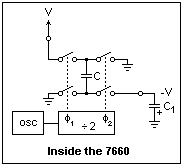At the right is shown the insides of an ICL7660 or the more recent LTC1044, a switched-capacitor circuit designed to provide a negative supply, but with many other uses. The flying capacitor C is charged one way, then discharged the other into a storage capacitor C1, which actually supplies the load when C is busy elsewhere. There is an oscillator and divide-by-two circuit that produce signals φ1 and φ2, which are nonoverlapping square waves of opposite phase. These signals operate the CMOS switches, so that the two switches on any one lead are never closed at the same time.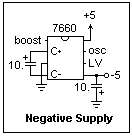The diagram at the left shows a -5 V supply that you can build and test. Three of the pins are not used. LV is only used for supply voltages less than 3 V, and a capacitor can be connected to OSC to slow down the oscillator, but why do that? "Boost" has uses for which you should consult the data sheets. It is not necessary to connect these pins in this application. The circuit is shown with 10 μF capacitors, which are a reasonable choice. Test the circuit with various load resistors, say 10k, 1k and 470. You'll find that it supplies about 10 mA before the voltage rises to around -4.5 V, plenty for CMOS chips and op-amps. Leave the circuit built--it will be needed later.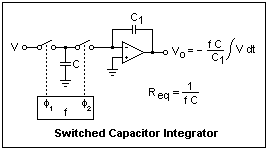For the next application of switched capacitors, consider the circuit shown at the right. It is an integrator, which adds up or integrates the input voltage. If the input is DC, it just keeps going until the op-amp saturates, but for an alternating input, the output can stay bounded. Note the same two-phase clock that alternately connects C to the source V and to the summing junction of the op-amp, which its output holds at ground by transferring all the charge that enters it to the integrating capacitor C1. The current is, on the average, i = fCV, so the switched capacitor acts like a resistance 1/fC. The beauty of this is that the effective resistance can be changed by changing the frequency.

Active filters can be made from such integrators, and the parameters of such a filter can be changed by changing the clock frequency. These are called state-variable filters. A second-order filter consists of a summer and two integrators in a loop (see the diagram below). The clock frequency is chosen much higher than the highest signal frequency. Usually, the clock and the signal can be separated well enough by a simple RC filter. The MF10 switched capacitor filter contains enough to make two double-pole filters of various types. There is quite a bit of flexibility, arranged by choosing the voltages applied to control inputs, but we shall concentrate on only one mode of operation out of six, with several sub-modes. Mode 1, which we shall study, can give us lowpass, bandpass and notch filters, depending on which output we choose. The properties of the filter are selected by three resistors, R1, R2 and R3, and the frequency of the square-wave clock fc. The pole frequency foof the filter is the clock frequency divided by 50 or 100. The Q of the filter is the ratio R3/R2, and its corner frequency fc for the lowpass filter is given by fc = fo sqrt{(1 - 1/2Q2) + sqrt[(1 - 1/2Q2)2 + 1]}.The connections for a Mode 1 filter are shown at the left, where we have selected a lowpass filter, taking the output from the LP pin. All the resistors are 10k, giving a Q of 1, as well as a unity gain at low frequencies, which is given by the familiar formula -R2/R1. If we desired a different Q or a different low-frequency gain, the resistors would be chosen appropriately (usually greater than 10k). The 50/100 pin is connected to +5, which gives the 50 divisor. Connecting it to ground gives 100. Note carefully that the power supply is bipolar, ±5 V. The -5 V can be supplied by the 7660 converter you just built. The MF10 has a maximum supply voltage of ±7 V, so it is wise to use no more than 6 V. It can be operated with a single supply, but this is so hard to do that it is best not to try. There are two supply pins for each polarity, one for the analog and one for digital. This is not so different voltages can be used (doing so will result in disaster), but so they can be separately bypassed.

The clock can be supplied by a relaxation oscillator made with a 74HC14, described in the unit on Relaxation Oscillators. If you use 470pF and 24k, the oscillator frequency will be about 120 kHz, giving fo = 2400 Hz. Since Q = 1, we expect fc = 1.272 fo or 3053 Hz. Using the oscilloscope, measure the input and output peak-to-peak voltages for frequencies between 100 Hz and 10kHz, and plot the gain in dB = 20 log (Vout/Vin) against log f. The curve will be flat out to the region of the corner frequency, where it will rise slightly, then bend over to fall off at a rate of -40 dB per decade of frequency, typical of a second-order filter. Extrapolate the linear falloff back to 0 dB to estimate the corner frequency, and compare with the calculated value.

Put a different capacitor in the oscillator, say 680pF or 330pF, and repeat enough gain measurements to convince yourself that the corner frequency has moved along with the clock frequency. This is the greatest advantage of the switched-capacitor filter. There are disadvantages, of course. One is the extra high frequency around for the clock. It gets in everywhere, and can be detected in the output. However, it is easily removed by an RC filter, so is really no problem. Another problem arises when the input has energy in the frequencies around the clock frequency (which it should not, since you should have filtered things like this out). An effect like Moiré fringes occurs, called aliasing, which may translate the energy down to lower frequencies and confuse the real signal. A final disadvantage is a rather limited dynamic range, because of all the op-amps involved and other things. Nevertheless, switched capacitor filters offer advantages found nowhere else!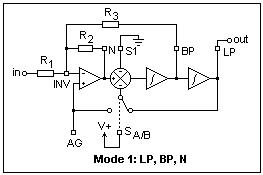Bandpass and notch filters can be realized simply by using the corresponding output pins, BP and N. The bandwidth is fo/Q, so a higher Q is necessary for a sharp passband or a sharp rejection band. The center frequency of these filters is just fo. A highpass filter is also available, but here the switch SA/B is connected to V-. The internal circuit for Mode 1 is shown at the right, and the switch can be seen. When it is thrown to the left, the highpass function can be created, with output at N. There is an additional R4 from INV to LP in this case, Mode 3, and fo and Q are multiplied by sqrt(R2/R4). The internal circuit is shown below.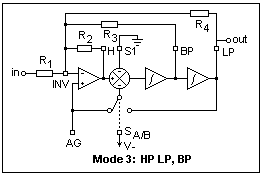In Mode 1, the input can be put into the low-impedance input S1, which should be driven by a source with less than 1k internal resistance. The low-frequency gain then becomes 1, and noninverting.

A fourth-order filter can be made by cascading the two filters in the MF10. It is necessary to arrange the gains and pole locations of the two stages properly to achieve the desired result, and to minimize level problems. Useful pointers are given in Horowitz and Hill, Chapter 5.